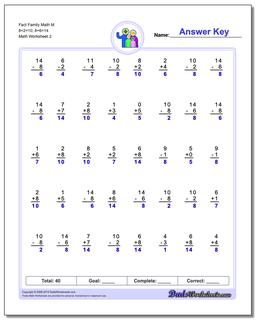One Dad. Four daughters. 9,211 worksheets... and counting!Fact Family Math M 8+2=10, 8+6=14 (Second Worksheet)

PropertyValue
DescriptionFact Family Math M 8+2=10, 8+6=14: These 40 problem addition and subtraction fact family worksheets are perfect for either quick practice or a one-minute timed test. Be sure to check out the stand-alone addition worksheets and subtraction worksheets for help on specific operations. (Second Worksheet)
Resource TypeWorksheet